# MA2051 - Ordinary Differential EquationsD96 Project #2A - Nonlinear Shocks

You have seen in lecture and exercises how to model a
spring-mass system with damping and external forcing.
One application for the model is an
automobile suspension system, where the forcing term is the road
(with or without frost heaves) and  shock absorbers provide
the damping.

In this project, you will study the behavior of the solution with different types of damping terms. Recognize that once you allow the damping coefficient to depend on the solution, the equation is no longer linear and most analytic solution methods (such as characteristic equations and undetermined coefficients) are useless. You have no choice but to study the system through numerical methods.

1. To start, describe the limiting behavior of the standard model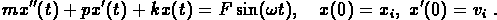How does the amplitude of the limiting solution depend on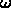? How does it depend on the initial data?

Compare your results with the graphs on page 280 and the formula given in Exercise 12 on page 282 in the text.

2. One of the best ways to analyze the solution curves for a second-order equation is to graph them in the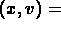(position, velocity) plane. Derive a system of first-order differential equations forand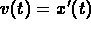and plot solution curves in theplane. Pay particular attention to the limiting behavior of the solutions. How does the limit depend on the initial data? How does it depend on other parameters in the model?

3. Mathematicians and engineers at the Renault Automobile Company have studied a new type of shock absorber which controls the damping coefficient. Their new shock absorber can increase the damping coefficient whenever the mass (i.e., the car) is returning toward equilibrium. In the mathematical model, the damping coefficient is a discontinuous function of bothand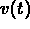.

Do some numerical experiments with the Renault shock absorber. Explain in detail how you program the damping coefficient in your equation. Takefor the forcing function and describe the limiting behavior of the solution for a range ofvalues. Does the new shock absorber give a "smoother" ride than the original shock absorber? Do you think that Renault should pursue its development of this new shock absorber?

4. Design your own shock absorber and analyze the resulting system of differential equations. Can yo do better then Renault?

```© 1996 by Will Brother.
All rights Reserved. File last modified on April 25, 1996.

```